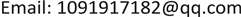1. 引言

2. 文献综述2.1. 养老保险与储蓄率问题

2.2. 人口年龄结构和储蓄率的问题

2.3. 住房价格与储蓄率的问题

3. 理论回顾

4. 模型分析4.1. 数据描述与变量选择

4.2. 城镇居民储蓄率的影响因素研究

The name and meaning of variable

s城镇居民储蓄率(%)
l o e城镇居民基本养老保险支出(亿元)的对数
l h e城镇居民医疗保险支出(亿元)的对数
l i n c城镇居民人均可支配收入的对数
n r一年期存款利率(名义利率%)

t时间(1989~2015分别记为1~27)

τ = ρ ^ S ( ρ ^ ) ，其中 S ( ρ ^ ) 为参数， ρ 的样本标准差。当统计量小于 τ 临界值时，拒绝原假设，可认为序列是平稳的。

s = − 89.114 + 38.282 l i n c − 0.330 n r − 2.827 l h e − 7.368 p − 3.748 l o e

( 22.223 )   ( 10.936 )   ( 0.193 )   ( 1.206 )   ( 1.291 )   ( 5.340 )

F = 251.049 ,   D . W . = 2.449 ,   R 2 = 0.984

Extracting variable’s trend components (α = 0.05

s = 13.030 + 0.702 te s显著显著0.9691
l o e = 2.212 + 0.084 te l o e显著显著0.976
h e = − 0.231 + 0.174 te l h e显著显著0.956
l i n c = 3.171 + 0.051 te l i n c显著显著0.983
n r = 9.321 − 0.318 te n r显著显著0.586
p = 1.158 + 0.071 te p显著显著0.892

The ADF test results of residual

e s第一种类型−4.252e s ∼ I ( 0 )
e l o e第一种类型−2.516e l o e ∼ I ( 0 )
e l h e第一种类型−2.183e l h e ∼ I ( 0 )
e l i n c第一种类型−4.431e l i n c ∼ I ( 0 )
e n r第一种类型−2.315e n r ∼ I ( 0 )
e p第一种类型−3.599e p ∼ I ( 0 )

5. 结论与建议